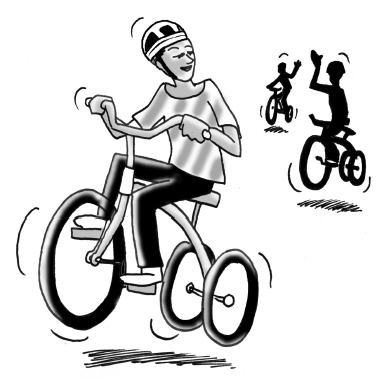### Home > CCA > Chapter 2 > Lesson 2.2.2 > Problem2-61

2-61.

THE BIG RACE − HEAT 3

Barbara, Mark, and Carlos participated in the third heat of “The Big Race.” Barbara thought she could win with a $3$ meter head start even though she only pedaled $3$ meters every $2$ seconds. Mark began at the starting line and finished the $20$ meter race in $5$ seconds. Meanwhile, Carlos rode his tricycle so that his distance ($y$) from the starting line in meters could be represented by the equation $y=\frac{5}{2}x+1$, where $x$ represents time in seconds.1. What is the dependent variable? What is the independent variable?

Between time and distance in this race, which variable changes? Which stays the same?

1. Using the given information, graph lines for Barbara, Mark, and Carlos on the same set of axes. Who won the $20$ meter race and will advance to the final race?

Every $2$ seconds, Barbara will go up $3$ meters. At $5$ seconds, Mark will be done with the $20$ meters. Use these clues as well as the equation given for Carlos to make a graph.

2. Find equations that describe Barbara’s and Mark’s motion.

The slope is the distance over the time (given) and the $y$-intercept is where they were when the race began, at $0$ seconds. (Remember Barbara had a head start).

$y=4x$

3. How fast did Carlos pedal? Write your answer as a unit rate.

If the slope is distance over time, what does the slope in the given equation tell us about Carlos' speed?

$5$ meters every $2$ seconds
Now write this as a unit rate.

• On the graph, at what point does Carlos' line cross Barbara's line? To confirm it algebraically, set the two equations equal to each other and solve for time $x$.